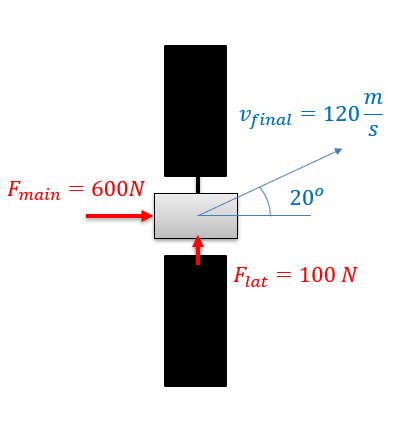﻿ Mechanics Map - The Equations of Motion with Rectangular Coordinates

# The Equations of Motion with Rectangular Coordinates

To start our discussion of kinetics in two dimensions, we will examine Newton's Second Law as applied to a fixed coordinate system. In its basic form, Newton's Second Law states that the sum of the forces on a body will be equal to mass of that body times the rate of acceleration. For bodies in motion, we can write this relationship out as the equation of motion.

 $\sum \vec{F}=m*\vec{a}$

With rectangular coordinates in two dimensions, we will break this single vector equation into two separate scalar equations. To solve the equations, we simply break any given forces and accelerations down into x and y components using sines and cosines and plug those known values in. With two equations, we should be able to solve for up to two unknown force or acceleration terms.

 $\sum F_{x}=m*a_{x}=m*\ddot{x}$ $\sum F_{y}=m*a_{y}=m*\ddot{y}$

Just as with a single dimension, the equations of motion are often used in conjunction with the kinematics equations, which relate positions, velocities and accelerations as discussed in the previous chapter. Depending on the problem being examined, the kinematics equations may need to be examined either before or after the kinetics equations.

Rectangular coordinates can be used in any kinetics problem, however they work best with problems where the forces do not change direction over time. Projectile motion is a good example of this, because the gravity force will maintain a constant direction, as opposed to the thrust force on a turning plane, where the thrust force changes direction with the plane.

## Worked Problems:

### Question 1:

You are controlling a satellite with a mass of 300 kg. The main and lateral thrusters can exert the forces shown. How long do you need to run each of the thrusters to achieve the final velocity as shown in the diagram? Assume the satellite has zero initial velocity.### Question 2:

A man in a flatbed truck starts from rest up a hill at an angle of 10 degrees. If he is carrying a 600 kg crate in the back and the static coefficient of friction is .3, what is the rate of acceleration at which we would expect the crate to begin sliding off of the back of the truck? How long will it take the truck to reach a speed of 25 m/s at this rate?